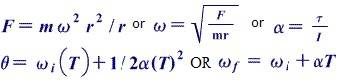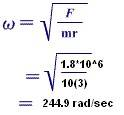# Rotational Equilibrium and Rotational Dynamics

## Homework Statement

Question Details:
A 10 kg engine is rotating at the rate of 20 rad/sec about a point on a wire 3 meters in length with a working tensile strength of 1.8*106 N. The engine is fired and produces an acceleration of 1 rad/sec2. What's the maximum possible ω (angular velocity) (in other words: 1) whats's the fastest ω which doesn't break the wire?) 2)How long should the engine be fired to reach the maximum possible ω? 3)How far will the engine travel in meters while it's accelerating to the maximum possible ω?

## Homework Equations## The Attempt at a Solution

I believe it's right, however this is only #1. Need help on #2 and #3.Thanks!

$$w_f = w_i + \alpha t$$